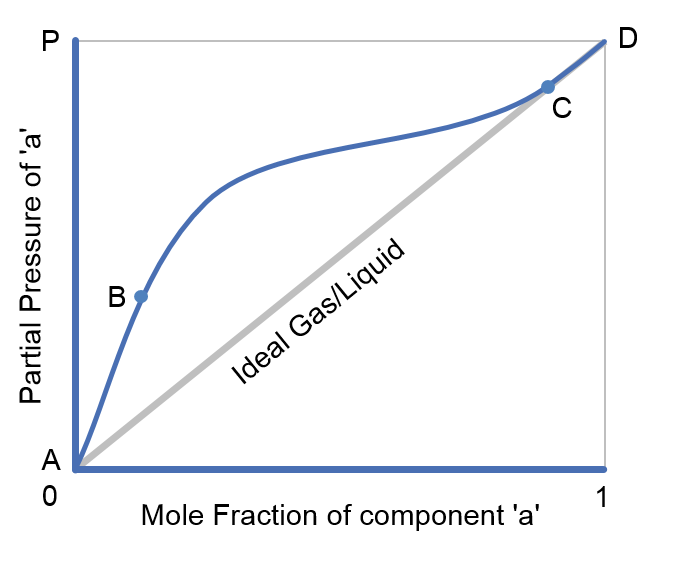f
Neutrium

# Properties

Relative Volatility and Activity CoefficientsRelative volatility is a comparative measure of the vapour pressures of components in a liquid mixture. It is commonly used in the design of absorption and separation processes such as distillation as it allows the difficulty of separating components to be quickly assessed.

Henry's LawHenry’s Law describes the relationship between the solubility of a gas in a liquid and the partial pressure of that gas above the liquid surface. A range of experimentally determined Henry’s constants are tabulated and can be used to determine the solubility of various gas species in water.

Raoult's LawRaoult’s law gives a method of estimating the composition and pressure of the vapour above a liquid mixture. This article describes the basis of Raoult’s law and provides an example of how to apply it.

Dalton's Law

Dalton’s law provides a method by which the total pressure of a gas mixture can be calculated using the partial pressures of the component gases of the mixture.

Wobbe IndexThe Wobbe Index is a measure of the interchangeability of fuel gases and their relative ability to deliver energy. It gives an indication of whether a turbine or burner will be able to run on an alternative fuel source without tuning or physical modifications.

Speed of Sound in Fluids and Fluid in Pipes

This article provides the formulae for the calculation of speed of sound in fluids and fluid filled circular pipes. The speed of sounds is important in piping systems for the calculation of choked flow for gases and pressure transient analysis of liquid filled systems.

Bulk Modulus of Elasticity of Liquids

The bulk modulus or elasticity is a measure of the resistance to compression of a substance. This article presents the bulk modulus for various liquids.

Specific Energy and Energy Density of Fuels

Specific energy and energy density are useful measures of the energy that will be released from a given weight or volume of fuel when it is burned. This article provides a quick reference for common values of specific energy and energy density.

Heat of Combustion

The heat of combustion is the energy liberated when a substance undergoes complete combustion, at constant pressure usually in an environment with excess Oxygen. The heat of combustion is utilised to quantify the performance of a fuel in a combustion system such as furnaces, power generation turbines and motors. This article describes the heat of combustion and provides a list of heats of combustion for commons fuels and fuel components.

Nominal Pipe Characteristics in Metric Units

Nominal Pipe Size (NPS) is a defined set of standard pipe sizes commonly used for process piping. NPS piping is defined in terms of a nominal diameter and wall thickness (defined by the pipe schedule). This article provides characteristics for NPS piping in metric units.